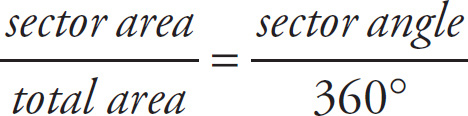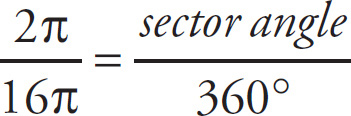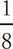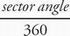# SAT Math Multiple Choice Question 148: Answer and Explanation

### Test Information

Question: 148

13. Circle O (not shown) is divided into three sectors. Points P, Q, and R are on the circumference of the circle. Sector POR has an area of 8π, and sector ROQ has an area of 6π. If the radius of circle O is 4, what is the measure of the central angle of sector QOP, in degrees?

• A. 45
• B. 90
• C. 135
• D. 180

A Since the radius of the circle is 4, the area of the entire circle is πr2 = π(42) = 16π. Sector POR has an area of 8π and sector ROQ has an area of 6π, so the remaining sector (QOP) has an area of 16π - 8π - 6π = 2π. You can set up a proportion to determine the associated angle using the following formula:. Using the numbers you now have, your calculation will look like this:or=. Multiply both sides of the equation by 360 to determine that the sector angle is 45°, which is (A).